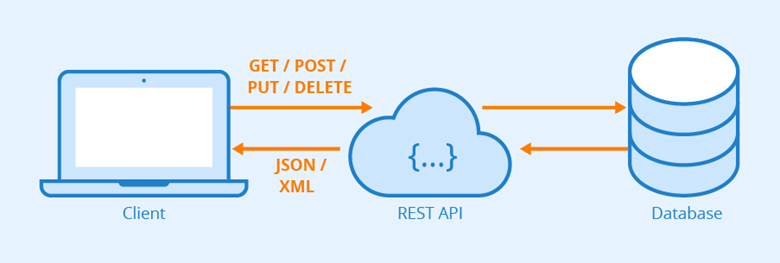# 航空公司客户价值分析模型LRFCM

## RFM模型的复习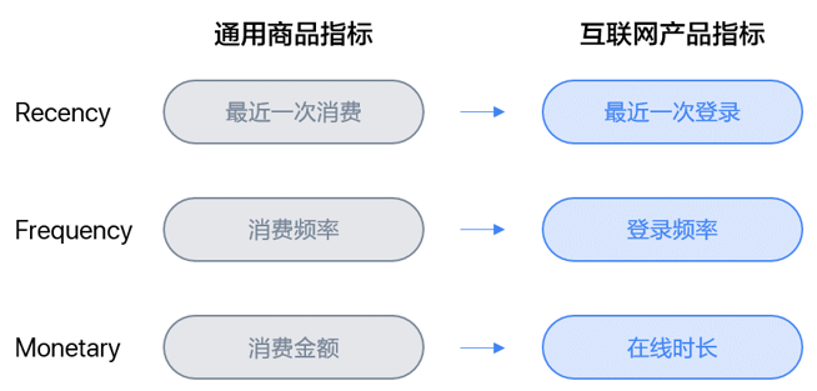## LRFCM模型简介

• L：客户关系长度。客户加入会员的日期至观测窗口结束日期的间隔。（反映可能的活跃时长）
• R：最近一次乘机时间。最近一次乘机日期至观测窗口结束日期的间隔。（反映当前的活跃状态）
• F：乘机频率。客户在观测窗口期内乘坐飞机的次数。（反映客户的忠诚度）
• M：飞行总里程。客户在观测窗口期内的飞行总里程。（反映客户对乘机的依赖性）
• C：平均折扣率。客户在观测窗口期内的平均折扣率。（舱位等级对应的折扣系数，侧面反映客户价值高低）

## LRFCM实战

• MEMBER_NO：会员卡号
• FFP_DATE：入会日期（办理会员卡的日期）
• FIRST_FLIGHT_DATE：第一次飞行日期
• GENDER：性别
• FFP_TIER：会员卡级别
• WORK_CITY：工作地所在城市
• WORK_PROVINCE：工作地所在身份
• WORK_COUNTRY：工作地所在身份
• AGE：年龄
• FLIGHT_COUNT：观测窗口内的飞行次数（频数）
• BP_SUM：观测窗口总基本积分（航空公里的里程就相当于积分，积累一定分数可以兑换奖品和免费里程）
• EP_SUM_YR_1：第一年精英资格积分
• EP_SUM_YR_2：第二年精英资格积分
• SUM_YR_1：第一年总票价
• SUM_YR_2：第二年总票价
• SEG_KM_SUM：观测窗口总飞行公里数
• WEIGHTED_SEG_KM：观测窗口总加权飞行公里数（Σ舱位折扣×航段距离）
• LAST_FLIGHT_DATE：最后一次飞行日期
• AVG_FLIGHT_COUNT：观测窗口季度平均飞行次数
• AVG_BP_SUM：观测窗口季度平均基本积分累积
• BEGIN_TO_FIRST：观察窗口内第一次乘机时间至MAX（观察窗口始端，入会时间）时长
• LAST_TO_END：最后一次乘机时间至观察窗口末端时长
• AVG_INTERVAL：平均乘机时间间隔
• MAX_INTERVAL：观察窗口内最大乘机间隔
• EXCHANGE_COUNT：积分兑换次数
• avg_discount：平均折扣率
• P1Y_Flight_Count：第1年乘机次数
• L1Y_Flight_Count：第2年乘机次数
• P1Y_BP_SUM：第1年里程积分
• L1Y_BP_SUM：第2年里程积分
• EP_SUM：观测窗口总精英积分
• Points_Sum：总累计积分
• L1Y_Points_Sum：第2年观测窗口总累计积分
• Ration_L1Y_Flight_Count：第2年的乘机次数比率
• Ration_P1Y_Flight_Count：第1年的乘机次数比率
• Ration_P1Y_BPS：第1年里程积分占最近两年积分比例
• Ration_L1Y_BPS：第2年里程积分占最近两年积分比例
• Point_NotFlight：非乘机的积分变动次数

1、数据准备

import pandas as pd
import numpy as np
from sklearn.cluster import KMeans
import matplotlib.pyplot as plt

# 观察各列数据
# explore = data.describe(percentiles=[], include='all').T
# explore['null'] = len(data) - explore['count']
# print(data.isnull().sum())

# 去除脏数据并只保留需要使用得字段
data_cleaned = data[data["SUM_YR_1"].notnull() & data["SUM_YR_2"].notnull()]
flag1 = data["SUM_YR_1"] != 0
flag2 = data["SUM_YR_2"] != 0
flag3 = (data["SEG_KM_SUM"] == 0) & (data["avg_discount"] == 0)
data_cleaned = data_cleaned[flag1 | flag2 | flag3]
data_cleaned = data_cleaned.reset_index(drop=True)
data_sepc = data_cleaned[['LOAD_TIME', 'FFP_DATE', 'LAST_TO_END', 'FLIGHT_COUNT', 'SEG_KM_SUM', 'avg_discount']]

# 将数据字段转换成LRFMC
data_sepc['FFP_DATE'] = pd.to_datetime(data_sepc['FFP_DATE'])
data_LRFMC = pd.DataFrame()
data_LRFMC['L'] = ((data_sepc['LOAD_TIME'] - data_sepc['FFP_DATE']) / np.timedelta64(1, 'D')) / 30
data_LRFMC['R'] = data_sepc['LAST_TO_END']
data_LRFMC['F'] = data_sepc['FLIGHT_COUNT']
data_LRFMC['M'] = data_sepc['SEG_KM_SUM']
data_LRFMC['C'] = data_sepc['avg_discount']

# 对LRFMC进行规格化处理
data_std_scale = (data_LRFMC - data_LRFMC.mean(axis=0)) / (data_LRFMC.std(axis=0))
data_std_scale.columns = ['Z'+i for i in data_std_scale.columns]


2、使用Kmeans进行聚类

def distEclud(vecA, vecB):
"""
计算两个向量的欧式距离的平方，并返回
"""
return np.sum(np.power(vecA - vecB, 2))

def test_Kmeans_nclusters(data_train):
"""
计算不同的k值时，SSE的大小变化
"""
data_train = data_train.values
nums = range(2, 10)
SSE = []
for num in nums:
sse = 0
kmodel = KMeans(n_clusters=num, n_jobs=4)
kmodel.fit(data_train)
cluster_ceter_list = kmodel.cluster_centers_
cluster_list = kmodel.labels_.tolist()
for index in range(len(data_train)):  # 计算残差平方和
cluster_num = cluster_list[index]
sse += distEclud(data_train[index, :], cluster_ceter_list[cluster_num])
print("簇数是", num, "时； SSE是", sse)
SSE.append(sse)
return nums, SSE

# 画图，通过观察SSE与k的取值尝试找出合适的k值
nums, SSE = test_Kmeans_nclusters(data_std_scale)
plt.rcParams['font.size'] = 12.0
plt.style.use('ggplot')
fig = plt.figure(figsize=(10, 10))
ax = fig.add_subplot(1, 1, 1)
ax.plot(nums, SSE, marker="+")
ax.set_xlabel("n_clusters", fontsize=18)
ax.set_ylabel("SSE", fontsize=18)
fig.suptitle("KMeans", fontsize=20)
plt.show()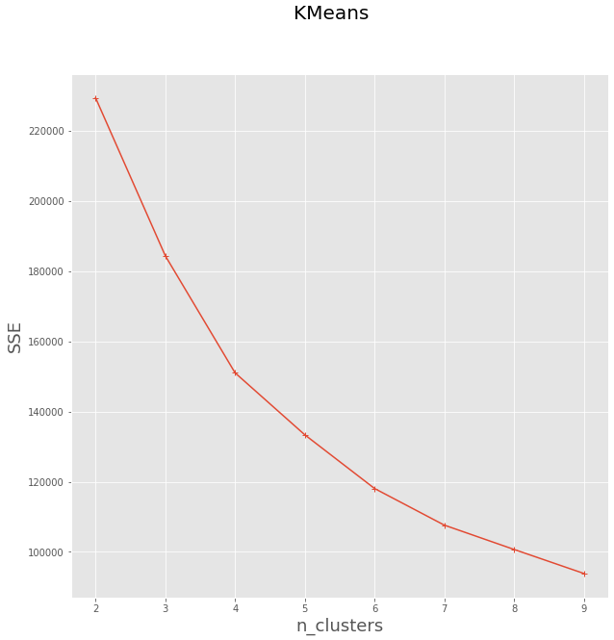# 获取聚类结果
k = 5
kmodel = KMeans(k)
kmodel.fit(data_std_scale)
r = pd.concat([data_std_scale, pd.Series(kmodel.labels_, index=data_std_scale.index)], axis=1)
r.columns = list(data_std_scale.columns) + ['mem_class']

# 获取聚类中心点结果
r1 = pd.Series(kmodel.labels_).value_counts()  # 统计各个类别的数目
r2 = pd.DataFrame(kmodel.cluster_centers_)  # 找出聚类中心
max_v = r2.values.max()
min_v = r2.values.min()
r_center = pd.concat([r2, r1], axis=1)  # 横向连接（0是纵向），得到聚类中心对应的类别下的数目
r_center.columns = list(data_std_scale.columns) + ['mem_class']
# mem_class = r.groupby('mem_class').size().reset_index(name='counts')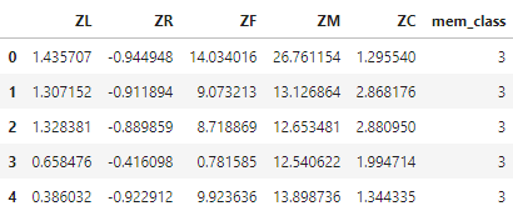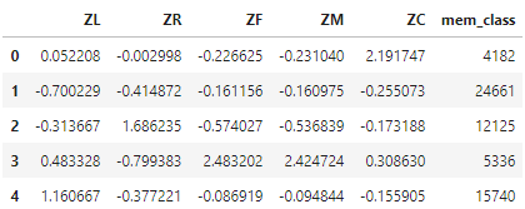3、对聚类结果进行可是化展现

# 查看每个类别下，每个数值得分布数据
for i in range(k):
data_std_scale[r['mem_class'] == i].plot(kind='kde', linewidth=2, subplots=True, sharex=False,
layout=(1, data_std_scale.shape), figsize=(16, 2))
# data_std_scale[r['mem_class'] == i].plot(kind='hist', linewidth=2, subplots=True, sharex=False,
#                                          layout=(1, data_std_scale.shape), figsize=(16, 2))
plt.legend()
plt.show()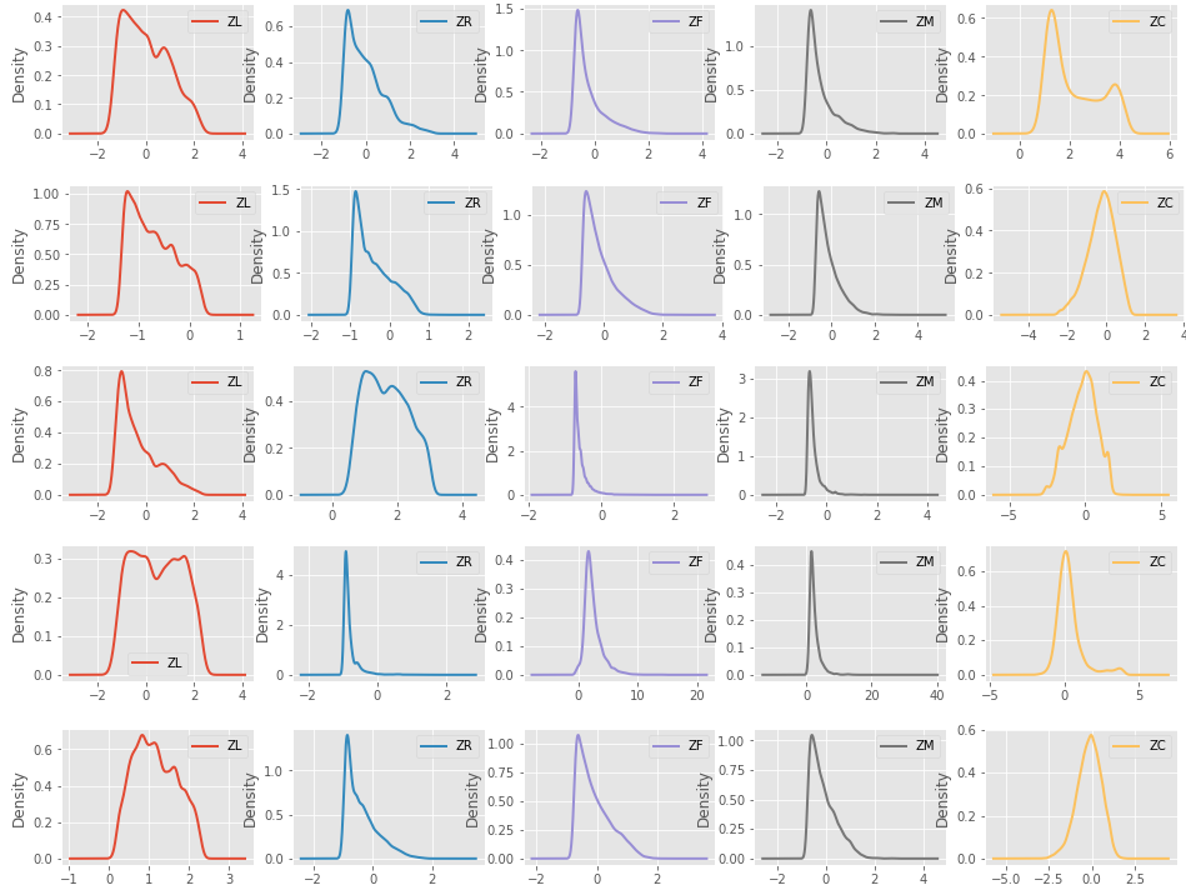# 将各个类别中心点值在同一图形上展示（便于对比）
clu = kmodel.cluster_centers_
feature = ['L', 'R', 'F', 'M', 'C']
colors = ['red', 'green', 'yellow', 'blue', 'black']
for i in range(k):
plt.plot(feature, clu[i], label='clustre ' + str(i), linewidth=2, color=colors[i], marker='o')
plt.ylabel('values')
plt.show()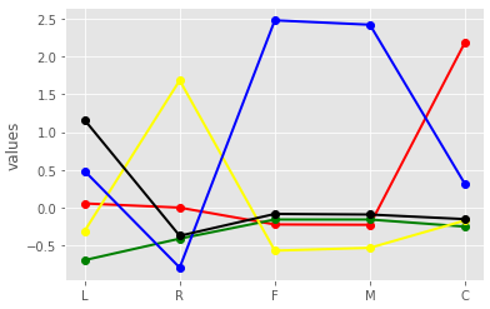# 以雷达图得方式展示（更加直观）
fig = plt.figure(figsize=(10, 8))
ax = fig.add_subplot(111, polar=True)
center_num = r_center.values
N = len(feature)
for i, v in enumerate(center_num):
# 设置雷达图的角度，用于平分切开一个圆面
angles = np.linspace(0, 2 * np.pi, N, endpoint=False)
# 为了使雷达图一圈封闭起来，需要下面的步骤
center = np.concatenate((v[:-1], [v]))
angles = np.concatenate((angles, [angles]))
# 绘制折线图
ax.plot(angles, center, 'o-', linewidth=2, label="category_%d : %d" % (i + 1, v[-1]))
# 填充颜色
ax.fill(angles, center, alpha=0.25)
# 添加每个特征的标签
ax.set_thetagrids(angles * 180 / np.pi, feature, fontsize=15)
# 设置雷达图的范围
ax.set_ylim(min_v - 0.1, max_v + 0.1)
# 添加标题
plt.title('LRFCM', fontsize=20)
# 添加网格线
ax.grid(True)
# 设置图例
plt.legend(loc='upper right', bbox_to_anchor=(1.3, 1.0), ncol=1, fancybox=True, shadow=True)
# 显示图形
plt.show()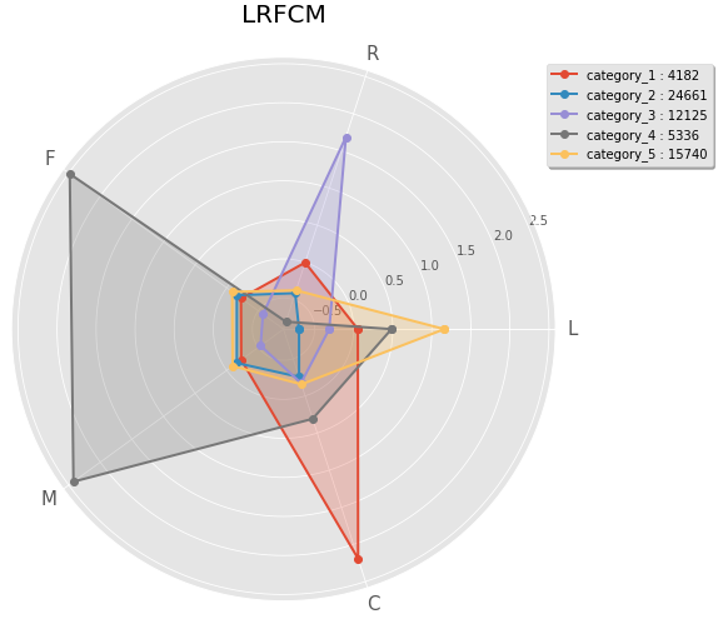4、对聚类结果进行分析及定义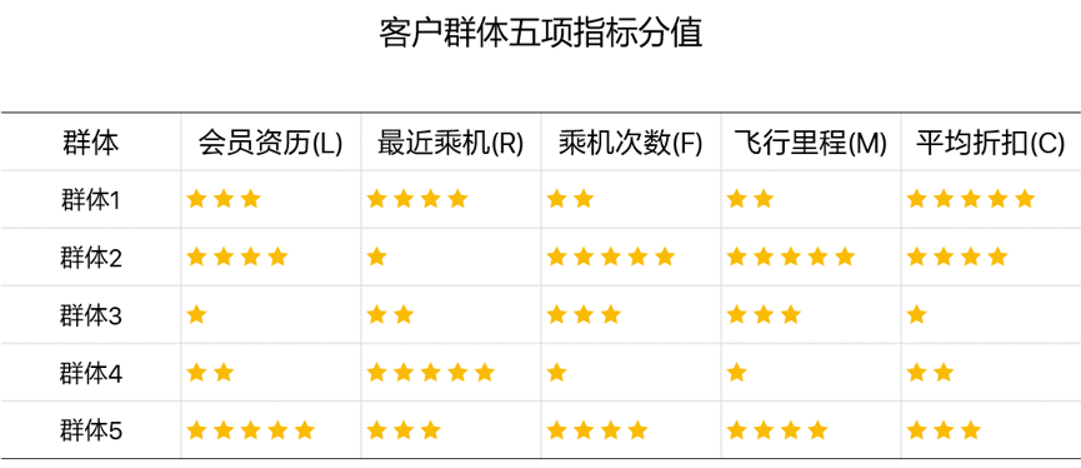• 重要保持客户：
• 平均折扣率高(C↑)，最近有乘机记录(R↓)，乘机次数高(F↑)或里程高(M↑)
• 这类客户机票票价高，不在意机票折扣，经常乘机，是最理想的客户类型
• 公司应优先将资源投放到他们身上，维持这类客户的忠诚度
• 重要发展客户
• 平均折扣率高(C↑)，最近有乘机记录(R↓)，乘机次数低(F↓)或里程低(M↓)
• 这类客户机票票价高，不在意机票折扣，最近有乘机记录，但总里程低，具有很大的发展潜力
• 公司应加强这类客户的满意度，使他们逐渐成为忠诚客户
• 重要挽留客户
• 平均折扣率高(C↑)，乘机次数高(F↑)或里程高(M↑)，最近无乘机记录(R↑)
• 这类客户总里程高，但较长时间没有乘机，可能处于流失状态
• 公司应加强与这类客户的互动，召回用户，延长客户的生命周期
• 一般客户
• 平均折扣率低(C↓)，最近无乘机记录(R↑)，乘机次数低(F↓)或里程低(M↓)，入会时间短(L↓)
• 这类客户机票票价低，经常买折扣机票，最近无乘机记录，可能是趁着折扣而选择购买，对品牌无忠诚度
• 公司需要在资源支持的情况下强化对这类客户的联系
• 低价值客户
• 平均折扣率低（C↓），最近无乘机记录（R↑），乘机次数低（F↓）或里程高（M↓），入会时间长（L↑）
• 这类客户与一般客户类似，机票票价低，经常买折扣机票，最近无乘机记录，可能是趁着折扣而选择购买，对品牌无忠诚度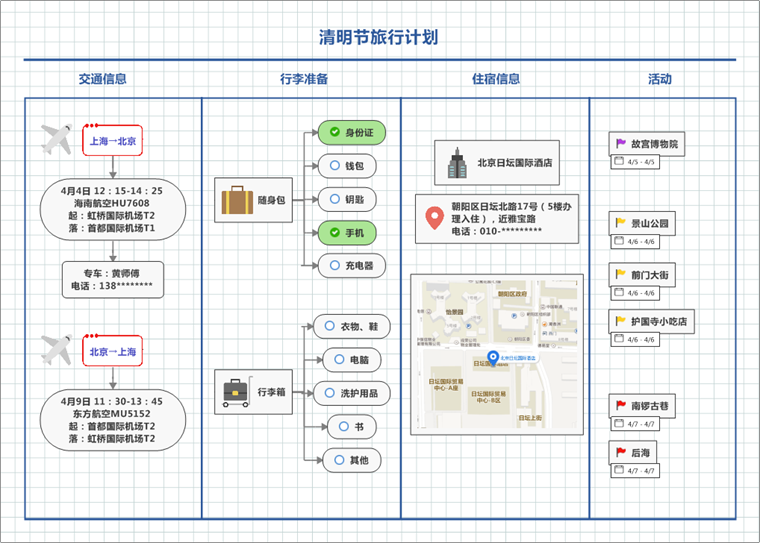##### 行程规划产品难在哪里？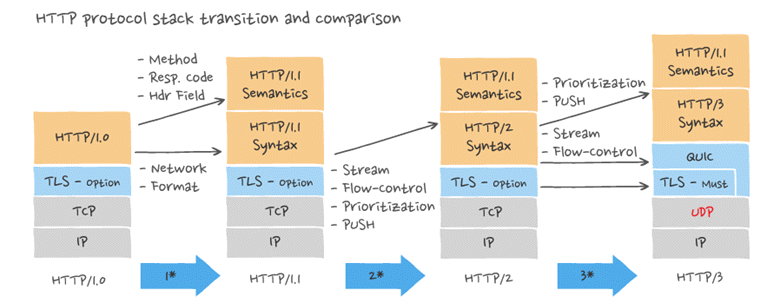##### HTTP协议演进与各版本特性